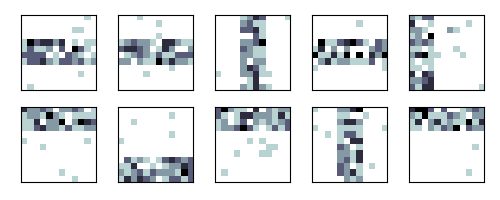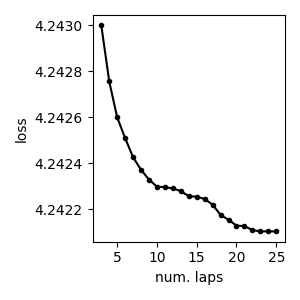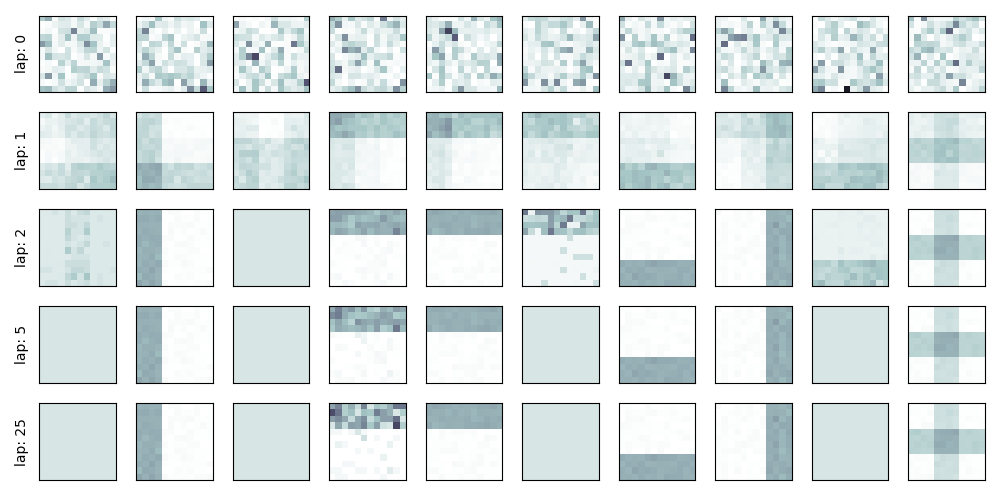# 01: Standard variational training for mixture model¶

How to train a mixture of multinomials.

import bnpy
import numpy as np
import os

from matplotlib import pylab
import seaborn as sns

FIG_SIZE = (3, 3)
SMALL_FIG_SIZE = (1,1)
pylab.rcParams['figure.figsize'] = FIG_SIZE


Read toy “bars” dataset from file.

dataset_path = os.path.join(bnpy.DATASET_PATH, 'bars_one_per_doc')
dataset = bnpy.data.BagOfWordsData.read_npz(
os.path.join(dataset_path, 'dataset.npz'))


Make a simple plot of the raw data

X_csr_DV = dataset.getSparseDocTypeCountMatrix()
bnpy.viz.BarsViz.show_square_images(
X_csr_DV[:10].toarray(), vmin=0, vmax=5)
#pylab.colorbar()
#pylab.clabel('word count')
pylab.tight_layout()Let’s do one single run of the VB algorithm.

Using 10 clusters and the ‘randexamples’ initializatio procedure.

trained_model, info_dict = bnpy.run(
dataset, 'FiniteMixtureModel', 'Mult', 'VB',
output_path='/tmp/bars_one_per_doc/helloworld-K=10/',
nLap=1000, convergeThr=0.0001,
K=10, initname='randomlikewang',
gamma0=50.0, lam=0.1)


Out:

WARNING: Found unrecognized keyword args. These are ignored.
--gamma0
Dataset Summary:
BagOfWordsData
size: 2000 units (documents)
vocab size: 144
min    5%   50%   95%   max
38    42    46    51    57  nUniqueTokensPerDoc
100   100   100   100   100  nTotalTokensPerDoc
Hist of word_count across tokens
1      2      3    <10   <100  >=100
0.38   0.29   0.19   0.14      0      0
Hist of unique docs per word type
<1    <10   <100  <0.10  <0.20  <0.50 >=0.50
0      0      0      0      0   >.99      0
Allocation Model:  Finite mixture model. Dir prior param 1.00
Obs. Data  Model:  Multinomial over finite vocabulary.
Obs. Data  Prior:  Dirichlet over finite vocabulary
lam = [0.1 0.1] ...
Initialization:
initname = randomlikewang
K = 10 (number of clusters)
seed = 1607680
elapsed_time: 0.0 sec
Learn Alg: VB | task  1/1 | alg. seed: 1607680 | data order seed: 8541952
task_output_path: /tmp/bars_one_per_doc/helloworld-K=10/1
1/1000 after      0 sec. |    226.2 MiB | K   10 | loss  4.711444785e+00 |
2/1000 after      0 sec. |    226.2 MiB | K   10 | loss  4.244123622e+00 | Ndiff  270.283
3/1000 after      0 sec. |    226.2 MiB | K   10 | loss  4.242999256e+00 | Ndiff   17.646
4/1000 after      0 sec. |    226.2 MiB | K   10 | loss  4.242755538e+00 | Ndiff   11.500
5/1000 after      0 sec. |    226.2 MiB | K   10 | loss  4.242599251e+00 | Ndiff    6.980
6/1000 after      0 sec. |    226.2 MiB | K   10 | loss  4.242508901e+00 | Ndiff    4.291
7/1000 after      0 sec. |    226.2 MiB | K   10 | loss  4.242425981e+00 | Ndiff    3.722
8/1000 after      0 sec. |    226.2 MiB | K   10 | loss  4.242371327e+00 | Ndiff    3.025
9/1000 after      0 sec. |    226.2 MiB | K   10 | loss  4.242328843e+00 | Ndiff    2.275
10/1000 after      0 sec. |    226.2 MiB | K   10 | loss  4.242298419e+00 | Ndiff    0.979
11/1000 after      0 sec. |    226.2 MiB | K   10 | loss  4.242296009e+00 | Ndiff    0.423
12/1000 after      0 sec. |    226.2 MiB | K   10 | loss  4.242290695e+00 | Ndiff    0.588
13/1000 after      0 sec. |    226.2 MiB | K   10 | loss  4.242278821e+00 | Ndiff    0.812
14/1000 after      0 sec. |    226.2 MiB | K   10 | loss  4.242257185e+00 | Ndiff    0.771
15/1000 after      0 sec. |    226.2 MiB | K   10 | loss  4.242254419e+00 | Ndiff    0.214
16/1000 after      0 sec. |    226.2 MiB | K   10 | loss  4.242244922e+00 | Ndiff    0.468
17/1000 after      0 sec. |    226.2 MiB | K   10 | loss  4.242219919e+00 | Ndiff    0.534
18/1000 after      0 sec. |    226.2 MiB | K   10 | loss  4.242175380e+00 | Ndiff    0.753
19/1000 after      0 sec. |    226.2 MiB | K   10 | loss  4.242154460e+00 | Ndiff    0.628
20/1000 after      0 sec. |    226.2 MiB | K   10 | loss  4.242128894e+00 | Ndiff    0.395
21/1000 after      1 sec. |    226.2 MiB | K   10 | loss  4.242126836e+00 | Ndiff    0.218
22/1000 after      1 sec. |    226.2 MiB | K   10 | loss  4.242109815e+00 | Ndiff    0.529
23/1000 after      1 sec. |    226.2 MiB | K   10 | loss  4.242103791e+00 | Ndiff    0.091
24/1000 after      1 sec. |    226.2 MiB | K   10 | loss  4.242103790e+00 | Ndiff    0.001
25/1000 after      1 sec. |    226.2 MiB | K   10 | loss  4.242103790e+00 | Ndiff    0.000
... done. converged.


First, we can plot the loss function over time We’ll skip the first few iterations, since performance is quite bad.

pylab.figure(figsize=FIG_SIZE)
pylab.plot(info_dict['lap_history'][2:], info_dict['loss_history'][2:], 'k.-')
pylab.xlabel('num. laps')
pylab.ylabel('loss')
pylab.tight_layout()Setup: Useful function to display learned bar structure over time.

def show_bars_over_time(
task_output_path=None,
query_laps=[0, 1, 2, 5, None],
ncols=10):
'''
'''
nrows = len(query_laps)
fig_handle, ax_handles_RC = pylab.subplots(
figsize=(SMALL_FIG_SIZE * ncols, SMALL_FIG_SIZE * nrows),
nrows=nrows, ncols=ncols, sharex=True, sharey=True)
for row_id, lap_val in enumerate(query_laps):
cur_model, lap_val = bnpy.load_model_at_lap(task_output_path, lap_val)
cur_topics_KV = cur_model.obsModel.getTopics()
# Plot the current model
cur_ax_list = ax_handles_RC[row_id].flatten().tolist()
bnpy.viz.BarsViz.show_square_images(
cur_topics_KV,
vmin=0.0, vmax=0.06,
ax_list=cur_ax_list)
cur_ax_list.set_ylabel("lap: %d" % lap_val)
pylab.tight_layout()


Show the clusters over time

show_bars_over_time(info_dict['task_output_path'])Total running time of the script: ( 0 minutes 2.685 seconds)

Gallery generated by Sphinx-Gallery